We are working to support a site-wide PDF but it is not yet available. You can download PDFs for individual lectures through the download badge on each lecture page.

Code should execute sequentially if run in a Jupyter notebook

Stackelberg Plans¶

In addition to what’s in Anaconda, this lecture will need the following libraries

In :
!pip install quantecon


Overview¶

This notebook formulates and computes a plan that a Stackelberg leader uses to manipulate forward-looking decisions of a Stackelberg follower that depend on continuation sequences of decisions made once and for all by the Stackelberg leader at time $0$

To facilitate computation and interpretation, we formulate things in a context that allows us to apply linear optimal dynamic programming

From the beginning we carry along a linear-quadratic model of duopoly in which firms face adjustment costs that make them want to forecast actions of other firms that influence future prices

Duopoly¶

Time is discrete and is indexed by $t = 0, 1, \ldots$

Two firms produce a single good whose demand is governed by the linear inverse demand curve

$$p_t = a_0 - a_1 (q_{1t}+ q_{2t} )$$

where $q_{it}$ is output of firm $i$ at time $t$ and $a_0$ and $a_1$ are both positive

$q_{10}, q_{20}$ are given numbers that serve as initial conditions at time $0$

By incurring a cost of change

$$\gamma v_{it}^2$$

where $\gamma > 0$, firm $i$ can change its output according to

$$q_{it+1} = q_{it} + v_{it}$$

Firm $i$‘s profits at time $t$ equal

$$\pi_{it} = p_t q_{it} - \gamma v_{it}^2$$

Firm $i$ wants to maximize the present value of its profits

$$\sum_{t=0}^\infty \beta^t \pi_{it}$$

where $\beta \in (0,1)$ is a time discount factor

Each firm $i=1,2$ chooses a sequence $\vec q_i \equiv \{q_{it+1}\}_{t=0}^\infty$ once and for all at time $0$

We let firm 2 be a Stackelberg leader and firm 1 be a Stackelberg follower

The leader firm 2 goes first and chooses $\{q_{2t+1}\}_{t=0}^\infty$ once and for all at time $0$

Knowing that firm 2 has chosen $\{q_{2t+1}\}_{t=0}^\infty$, the follower firm 1 goes second and chooses $\{q_{1t+1}\}_{t=0}^\infty$ once and for all at time $0$

In choosing $\vec q_2$, firm 2 takes into account that firm 1 will base its choice of $\vec q_1$ on firm 2’s choice of $\vec q_2$

Abstract statement of the leader’s and follower’s problems¶

We can express firm 1’s problem as

$$\max_{\vec q_1} \Pi_1(\vec q_1; \vec q_2)$$

where the appearance behind the semi-colon indicates thata $\vec q_2$ is given

Firm 1’s problem induces a best response mapping

$$\vec q_1 = B(\vec q_2)$$

(Here $B$ maps a sequence into a sequence)

$$\max_{\vec q_2} \Pi_2 (B(\vec q_2), \vec q_2)$$

whose maximizer is a sequence $\vec q_2$ that depends on the initial conditions $q_{10}, q_{20}$ and the parameters of the model $a_0, a_1, \gamma$

This formulation captures key features of the model

• Both firms make once-and-for-all choices at time $0$
• This is true even though both firms are choosing sequences of quantities that are indexed by time
• The Stackelberg leader chooses first within time $0$, knowing that the Stackelberg follower will choose second within time $0$

While our abstract formulation reveals the timing protocol and equilibrium concept well, it obscures details that must be addressed when we want to compute and interpret a Stackelberg plan and the follower’s best response to it

To gain insights about these things, we study them in more detail

Firms’ problems¶

Firm 1 acts as if firm 2’s sequence $\{q_{2t+1}\}_{t=0}^\infty$ is given and beyond its control

Firm 2 knows that firm 1 chooses second and takes this into account in choosing $\{q_{2t+1}\}_{t=0}^\infty$

In the spirit of working backwards, we study firm 1’s problem first, taking $\{q_{2t+1}\}_{t=0}^\infty$ as given

We can formulate firm 1’s optimum problem in terms of the Lagrangian

$$L=\sum_{t=0}^{\infty}\beta^{t}\{a_{0}q_{1t}-a_{1}q_{1t}^{2}-a_{1}q_{1t}q_{2t}-\gamma v_{1t}^{2}+\lambda_{t}[q_{1t}+v_{1t}-q_{1t+1}]\}$$

Firm 1 seeks a maximum with respect to $\{q_{1t+1}, v_{1t} \}_{t=0}^\infty$ and a minimum with respect to $\{ \lambda_t\}_{t=0}^\infty$

We approach this problem using methods described in Ljungqvist and Sargent RMT5 chapter 2, appendix A and Macroeconomic Theory, 2nd edition, chapter IX

First-order conditions for this problem are

\eqalign{ \frac{\partial L}{\partial q_{1t}} & = a_0 - 2 a_1 q_{1t} - a_1 q_{2t} + \lambda_t - \beta^{-1} \lambda_{t-1} = 0 , \quad t \geq 1 \cr \frac{\partial L}{\partial v_{1t}} & = -2 \gamma v_{1t} + \lambda_t = 0 , \quad t \geq 0 \cr }

These first-order conditions and the constraint $q_{1t+1} = q_{1t} + v_{1t}$ can be rearranged to take the form

\eqalign{ v_{1t} & = \beta v_{1t+1} + \frac{\beta a_0}{2 \gamma} - \frac{\beta a_1}{\gamma} q_{1t+1} - \frac{\beta a_1}{2 \gamma} q_{2t+1} \cr q_{t+1} & = q_{1t} + v_{1t} }

We can substitute the second equation into the first equation to obtain

$$(q_{1t+1} - q_{1t} ) = \beta (q_{1t+2} - q_{1t+1}) + c_0 - c_1 q_{1t+1} - c_2 q_{2t+1}$$

where $c_0 = \frac{\beta a_0}{2 \gamma}, c_1 = \frac{\beta a_1}{\gamma}, c_2 = \frac{\beta a_1}{2 \gamma}$

This equation can in turn be rearranged to become the second-order difference equation

$$q_{1t} + (1+\beta + c_1) q_{1t+1} - \beta q_{1t+2} = c_0 - c_2 q_{2t+1} \tag{1}$$

Equation (1) is a second-order difference equation in the sequence $\vec q_1$ whose solution we want

It satisfies two boundary conditions:

• an initial condition that $q_{1,0}$, which is given
• a terminal condition requiring that $\lim_{T \rightarrow + \infty} \beta^T q_{1t}^2 < + \infty$

Using the lag operators described in chapter IX of Macroeconomic Theory, Second edition (1987), difference equation (1) can be written as

$$\beta(1 - \frac{1+\beta + c_1}{\beta} L + \beta^{-1} L^2 ) q_{1t+2} = - c_0 + c_2 q_{2t+1}$$

The polynomial in the lag operator on the left side can be factored as

$$(1 - \frac{1+\beta + c_1}{\beta} L + \beta^{-1} L^2 ) = ( 1 - \delta_1 L ) (1 - \delta_2 L) \tag{2}$$

where $0 < \delta_1 < 1 < \frac{1}{\sqrt{\beta}} < \delta_2$

Because $\delta_2 > \frac{1}{\sqrt{\beta}}$ the operator $(1 - \delta_2 L)$ contributes an unstable component if solved backwards but a stable component if solved forwards

Mechanically, write

$$(1- \delta_2 L) = -\delta_{2} L (1 - \delta_2^{-1} L^{-1} )$$

and compute the following inverse operator

$$\left[-\delta_{2} L (1 - \delta_2^{-1} L^{-1} )\right]^{-1} = - \delta_2 (1 - {\delta_2}^{-1} )^{-1} L^{-1}$$

Operating on both sides of equation (2) with $\beta^{-1}$ times this inverse operator gives the follower’s decision rule for setting $q_{1t+1}$ in the feedback-feedforward form

$$q_{1t+1} = \delta_1 q_{1t} - c_0 \delta_2^{-1} \beta^{-1} \frac{1}{1 -\delta_2^{-1}} + c_2 \delta_2^{-1} \beta^{-1} \sum_{j=0}^\infty \delta_2^j q_{2t+j+1} , \quad t \geq 0 \tag{3}$$

The problem of the Stackelberg leader firm 2 is to choose the sequence $\{q_{2t+1}\}_{t=0}^\infty$ to maximize its discounted profits

$$\sum_{t=0}^\infty \beta^t \{ (a_0 - a_1 (q_{1t} + q_{2t}) ) q_{2t} - \gamma (q_{2t+1} - q_{2t})^2 \}$$

subject to the sequence of constraints (3) for $t \geq 0$

We can put a sequence $\{\theta_t\}_{t=0}^\infty$ of Lagrange multipliers on the sequence of equations (3) and formulate the following Lagrangian for the Stackelberg leader firm 2’s problem

\eqalign{ \tilde L & = \sum_{t=0}^\infty \beta^t\{ (a_0 - a_1 (q_{1t} + q_{2t}) ) q_{2t} - \gamma (q_{2t+1} - q_{2t})^2 \} \cr & + \sum_{t=0}^\infty \beta^t \theta_t \{ \delta_1 q_{1t} - c_0 \delta_2^{-1} \beta^{-1} \frac{1}{1 -\delta_2^{-1}} + c_2 \delta_2^{-1} \beta^{-1} \sum_{j=0}^\infty \delta_2^{-j} q_{2t+j+1} - q_{1t+1} \} } \tag{4}

subject to initial conditions for $q_{1t}, q_{2t}$ at $t=0$

Comments: We have formulated the Stackelberg problem in a space of sequences

The max-min problem associated with Lagrangian (4) is unpleasant because the time $t$ component of firm $1$‘s payoff function depends on the entire future of its choices of $\{q_{1t+j}\}_{j=0}^\infty$

This renders a direct attack on the problem cumbersome

Therefore, below, we will formulate the Stackelberg leader’s problem recursively

We’ll put our little duopoly model into a broader class of models with the same conceptual structure

The Stackelberg Problem¶

We formulate a class of linear-quadratic Stackelberg leader-follower problems of which our duopoly model is an instance

We use the optimal linear regulator (a.k.a. the linear-quadratic dynamic programming problem described in LQ Dynamic Programming problems) to represent a Stackelberg leader’s problem recursively

Let $z_t$ be an $n_z \times 1$ vector of natural state variables

Let $x_t$ be an $n_x \times 1$ vector of endogenous forward-looking variables that are physically free to jump at $t$

In our duopoly example $x_t = v_{1t}$, the time $t$ decision of the Stackelberg follower

Let $u_t$ be a vector of decisions chosen by the Stackelberg leader at $t$

The $z_t$ vector is inherited physically from the past

But $x_t$ is a decision made by the Stackelberg follower at time $t$ that is the follower’s best response to the choice of an entire sequence of decisions made by the Stackelberg leader at time $t=0$

Let

$$y_t = \begin{bmatrix} z_t \\ x_t \end{bmatrix}$$

Represent the Stackelberg leader’s one-period loss function as

$$r(y, u) = y' R y + u' Q u$$

Subject to an initial condition for $z_0$, but not for $x_0$, the Stackelberg leader wants to maximize

$$-\sum_{t=0}^\infty \beta^t r(y_t, u_t) \tag{5}$$

The Stackelberg leader faces the model

$$\begin{bmatrix} I & 0 \\ G_{21} & G_{22} \end{bmatrix} \begin{bmatrix} z_{t+1} \\ x_{t+1} \end{bmatrix} = \begin{bmatrix} \hat A_{11} & \hat A_{12} \\ \hat A_{21} & \hat A_{22} \end{bmatrix} \begin{bmatrix} z_t \\ x_t \end{bmatrix} + \hat B u_t \tag{6}$$

We assume that the matrix $\begin{bmatrix} I & 0 \\ G_{21} & G_{22} \end{bmatrix}$ on the left side of equation (6) is invertible, so that we can multiply both sides by its inverse to obtain

$$\begin{bmatrix} z_{t+1} \\ x_{t+1} \end{bmatrix} = \begin{bmatrix} A_{11} & A_{12} \\ A_{21} & A_{22} \end{bmatrix} \begin{bmatrix} z_t \\ x_t \end{bmatrix} + B u_t \tag{7}$$

or

$$y_{t+1} = A y_t + B u_t \tag{8}$$

Interpretation of the second block of equations¶

The Stackelberg follower’s best response mapping is summarized by the second block of equations of (7)

In particular, these equations are the first-order conditions of the Stackelberg follower’s optimization problem (i.e., its Euler equations)

These Euler equations summarize the forward-looking aspect of the follower’s behavior and express how its time $t$ decision depends on the leader’s actions at times $s \geq t$

When combined with a stability condition to be imposed below, the Euler equations summarize the follower’s best response to the sequence of actions by the leader

The Stackelberg leader maximizes (5) by choosing sequences ${u_t, x_t, z_{t+1}}\_{t=0}^{\infty}$ subject to (8) and an initial condition for $z_0$

Note that we have an initial condition for $z_0$ but not for $x_0$

$x_0$ is among the variables to be chosen at time $0$ by the Stackelberg leader

The Stackelberg leader uses its understanding of the responses restricted by (8) to manipulate the follower’s decisions

More mechanical details¶

For any vector $a_t$, define $\vec a_t = [a_t, a\_{t+1} \ldots ]$

Define a feasible set of $(\vec y_1, \vec u_0)$ sequences

$$\Omega(y_0) = \left\{ (\vec y_1, \vec u_0) : y_{t+1} = A y_t + B u_t, \forall t \geq 0 \right\}$$

Please remember that the follower’s Euler equation is embedded in the system of dynamic equations $y_{t+1} = A y_t + B u_t$

Note that in the definition of $\Omega(y_0)$, $y_0$ is taken as given

Although it is taken as given in $\Omega(y_0)$, eventually, the $x_0$ component of $y_0$ will be chosen by the Stackelberg leader

Two Subproblems¶

Once again we use backward induction

We express the Stackelberg problem in terms of two subproblems

Subproblem 1 is solved by a continuation Stackelberg leader at each date $t \geq 0$

Subproblem 2 is solved the Stackelberg leader at $t=0$

The two subproblems are designed

• to respect the protocol in which the follower chooses $\vec q_1$ after seeing $\vec q_2$ chosen by the leader
• to make the leader choose $\vec q_2$ while respecting that $\vec q_1$ will be the follower’s best response to $\vec q_2$
• to represent the leader’s problem recursively by artfully choosing the state variables confronting and the control variables available to the leader

Subproblem 1¶

$$v(y_0) = \max_{(\vec y_1, \vec u_0) \in \Omega(y_0)} - \sum_{t=0}^\infty \beta^t r(y_t, u_t)$$

Subproblem 2¶

$$w(z_0) = \max_{x_0} v(y_0)$$

Subproblem 1 takes the vector of forward-looking variables $x_0$ as given

Subproblem 2 optimizes over $x_0$

The value function $w(z_0)$ tells the value of the Stackelberg plan as a function of the vector of natural state variables at time $0$, $z_0$

Two Bellman equations¶

We now describe Bellman equations for $v(y)$ and $w(z_0)$

Subproblem 1¶

The value function $v(y)$ in subproblem 1 satisfies the Bellman equation

$$v(y) = \max_{u, y^*} \left\{ - r(y,u) + \beta v(y^*) \right\} \tag{9}$$

where the maximization is subject to

$$y^* = A y + B u$$

and $y^*$ denotes next period’s value

Substituting $v(y) = - y'P y$ into Bellman equation (9) gives

$$-y' P y = {\rm max}_{ u, y^*} \left\{ - y' R y - u'Q u - \beta y^{* \prime} P y^* \right\}$$

which as in lecture linear regulator gives rise to the algebraic matrix Riccati equation

$$P = R + \beta A' P A - \beta^2 A' P B ( Q + \beta B' P B)^{-1} B' P A$$

and the optimal decision rule coefficient vector

$$F = \beta( Q + \beta B' P B)^{-1} B' P A$$

where the optimal decision rule is

$$u_t = - F y_t$$

Subproblem 2¶

We find an optimal $x_0$ by equating to zero the gradient of $v(y_0)$ with respect to $x_0$:

$$-2 P_{21} z_0 - 2 P_{22} x_0 =0,$$

which implies that

$$x_0 = - P_{22}^{-1} P_{21} z_0$$

Stackelberg plan¶

Now let’s map our duopoly model into the above setup.

We we’ll formulate a state space system

$$y_t = \begin{bmatrix} z_t \cr x_t \end{bmatrix}$$

where in this instance $x_t = v_{1t}$, the time $t$ decision of the follower firm 1

Calculations to prepare duopoly model¶

Now we’ll proceed to cast our duopoly model within the framework of the more general linear-quadratic structure described above

That will allow us to compute a Stackelberg plan simply by enlisting a Riccati equation to solve a linear-quadratic dynamic program

As emphasized above, firm 1 acts as if firm 2’s decisions $\{q_{2t+1}, v_{2t}\}_{t=0}^\infty$ are given and beyond its control

Firm 1’s problem¶

We again formulate firm 1’s optimum problem in terms of the Lagrangian

$$L=\sum_{t=0}^{\infty}\beta^{t}\{a_{0}q_{1t}-a_{1}q_{1t}^{2}-a_{1}q_{1t}q_{2t}-\gamma v_{1t}^{2}+\lambda_{t}[q_{1t}+v_{1t}-q_{1t+1}]\}$$

Firm 1 seeks a maximum with respect to $\{q_{1t+1}, v_{1t} \}_{t=0}^\infty$ and a minimum with respect to $\{ \lambda_t\}_{t=0}^\infty$

First-order conditions for this problem are

\eqalign{ \frac{\partial L}{\partial q_{1t}} & = a_0 - 2 a_1 q_{1t} - a_1 q_{2t} + \lambda_t - \beta^{-1} \lambda_{t-1} = 0 , \quad t \geq 1 \cr \frac{\partial L}{\partial v_{1t}} & = -2 \gamma v_{1t} + \lambda_t = 0 , \quad t \geq 0 \cr }

These first-order order conditions and the constraint $q_{1t+1} = q_{1t} + v_{1t}$ can be rearranged to take the form

\eqalign{ v_{1t} & = \beta v_{1t+1} + \frac{\beta a_0}{2 \gamma} - \frac{\beta a_1}{\gamma} q_{1t+1} - \frac{\beta a_1}{2 \gamma} q_{2t+1} \cr q_{t+1} & = q_{1t} + v_{1t} }

We use these two equations as components of the following linear system that confronts a Stackelberg continuation leader at time $t$

$$\begin{bmatrix} 1 & 0 & 0 & 0 \cr 0 & 1 & 0 & 0 \cr 0 & 0 & 1 & 0 \cr \frac{\beta a_0}{2 \gamma} & - \frac{\beta a_1}{2 \gamma} & -\frac{\beta a_1}{\gamma} & \beta \end{bmatrix} \begin{bmatrix} 1 \cr q_{2t+1} \cr q_{1t+1} \cr v_{1t+1} \end{bmatrix} = \begin{bmatrix} 1 & 0 & 0 & 0 \cr 0 & 1 & 0 & 0 \cr 0 & 0 & 1 & 1 \cr 0 & 0 & 0 & 1 \end{bmatrix} \begin{bmatrix} 1 \cr q_{2t} \cr q_{1t} \cr v_{1t} \end{bmatrix} + \begin{bmatrix} 0 \cr 1 \cr 0 \cr 0 \end{bmatrix} v_{2t}$$

Time $t$ revenues of firm 2 are $\pi_{2t} = a_0 q_{2t} - a_1 q_{2t}^2 - a_1 q_{1t} q_{2t}$ which evidently equal

$$z_t' R_1 z_t \equiv \begin{bmatrix} 1 \cr q_{2t} \cr q_{1t} \end{bmatrix}' \begin{bmatrix} 0 & \frac{a_0}{2}& 0 \cr \frac{a_0}{2} & -a_1 & -\frac{a_1}{2}\cr 0 & -\frac{a_1}{2} & 0 \end{bmatrix} \begin{bmatrix} 1 \cr q_{2t} \cr q_{1t} \end{bmatrix}$$

If we set $Q = \gamma$, then firm 2’s period $t$ profits can then be written

$$y_t' R y_t - Q v_{2t}^2$$

where

$$y_t = \begin{bmatrix} z_t \cr x_t \end{bmatrix}$$

with $x_t = v_{1t}$ and

$$R = \begin{bmatrix} R_1 & 0 \cr 0 & 0 \end{bmatrix}$$

We’ll report results of implementing this code soon

But first we want to represent the Stackelberg leader’s optimal choices recursively

It is important to do this for several reasons:

• properly to interpret a representation of the Stackelberg leaders’s choice as a sequence of history-dependent functions
• to formulate a recursive version of the follower’s choice problem

First let’s get a recursive representation of the Stackelberg leader’s choice of $\vec q_2$ for our duopoly model

Recursive representation of Stackelberg plan¶

In order to attain an appropriate representation of the Stackelberg leader’s history-dependent plan, we will employ what amounts to a version of the Big K, little k device often used in macroeconomics by distinguishing $z_t$, which depends partly on decisions $x_t$ of the followers, from another vector $\check z_t$, which does not

We will use $\check z_t$ and its history $\check z^t = [\check z_t, \check z_{t-1}, \ldots, \check z_0]$ to describe the sequence of the Stackelberg leader’s decisions that the Stackelberg follower takes as given

Thus, we let $\check y_t' = \begin{bmatrix}\check z_t' & \check x_t'\end{bmatrix}$ with initial condition $\check z_0 = z_0$ given

That we distinguish $\check z_t$ from $z_t$ is part and parcel of the Big K, little k device in this instance

We have demonstrated that a Stackelberg plan for $\{u_t\}_{t=0}^\infty$ has a recursive representation

\eqalign{ \check x_0 & = - P_{22}^{-1} P_{21} z_0 \cr u_t & = - F \check y_t, \quad t \geq 0 \cr \check y_{t+1} & = (A - BF) \check y_t, \quad t \geq 0 }

From this representation we can deduce the sequence of functions $\sigma = \{\sigma_t(\check z^t)\}_{t=0}^\infty$ that comprise a Stackelberg plan

For convenience, let $\check A \equiv A - BF$ and partition $\check A$ conformably to the partition $y_t = \begin{bmatrix}\check z_t \cr \check x_t \end{bmatrix}$ as

$$\begin{bmatrix}\check A_{11} & \check A_{12} \cr \check A_{21} & \check A_{22} \end{bmatrix}$$

Let $H^0_0 \equiv - P_{22}^{-1} P_{21}$ so that $\check x_0 = H^0_0 \check z_0$

Then iterations on $\check y_{t+1} = \check A \check y_t$ starting from initial condition $\check y_0 = \begin{bmatrix}\check z_0 \cr H^0_0 \check z_0\end{bmatrix}$ imply that for $t \geq 1$

$$x_t = \sum_{j=1}^t H_j^t \check z_{t-j}$$

where

\eqalign{ H^t_1 & = \check A_{21} \cr H^t_2 & = \check A_{22} \check A_{21} \cr \ \ \vdots \ \ & \ \ \quad \vdots \cr H^t_{t-1} & = \check A_{22}^{t-2} \check A_{21} \cr H^t_t & = \check A_{22}^{t-1}(\check A_{21} + \check A_{22} H^0_0 ) }

An optimal decision rule for the Stackelberg’s choice of $u_t$ is

$$u_t = - F \check y_t \equiv - \begin{bmatrix} F_z & F_x \cr \end{bmatrix} \begin{bmatrix}\check z_t \cr x_t \cr \end{bmatrix}$$

or

$$u_t = - F_z \check z_t - F_x \sum_{j=1}^t H^t_j z_{t-j} = \sigma_t(\check z^t) \tag{10}$$

Representation (10) confirms that whenever $F_x \neq 0$, the typical situation, the time $t$ component $\sigma_t$ of a Stackelberg plan is history dependent, meaning that the Stackelberg leader’s choice $u_t$ depends not just on $\check z_t$ but on components of $\check z^{t-1}$

After all, at the end of the day, it will turn out that because we set $\check z_0 = z_0$, it will be true that $z_t = \check z_t$ for all $t \geq 0$

Then why did we distinguish $\check z_t$ from $z_t$?

The answer is that if we want to present to the Stackelberg follower a history-dependent representation of the Stackelberg leader’s sequence $\vec q_2$, we must use representation (10) cast in terms of the history $\check z^t$ and not a corresponding representation cast in terms of $z^t$

Dynamic programming and time consistency of follower’s problem¶

Given the sequence $\vec q_2$ chosen by the Stackelberg leader in our duopoly model, it turns out that the Stackelberg follower’s problem is recursive in the natural state variables that confront a follower at any time $t \geq 0$

This means that the follower’s plan is time consistent

To verify these claims, we’ll formulate a recursive version of a follower’s problem that builds on our recursive representation of the Stackelberg leader’s plan and our use of the Big K, little k idea

Recursive formulation of a follower’s problem¶

We now use what amounts to another “Big $K$, little $k$” trick (see rational expectations equilibrium) to formulate a recursive version of a follower’s problem cast in terms of an ordinary Bellman equation

Firm 1, the follower, faces $\{q_{2t}\}_{t=0}^\infty$ as a given quantity sequence chosen by the leader and believes that its output price at $t$ satisfies

$$p_t = a_0 - a_1 ( q_{1t} + q_{2t}) , \quad t \geq 0$$

Our challenge is to represent $\{q_{2t}\}_{t=0}^\infty$ as a given sequence

To do so, recall that under the Stackelberg plan, firm 2 sets output according to the $q_{2t}$ component of

$$y_{t+1} = \begin{bmatrix} 1 \cr q_{2t} \cr q_{1t} \cr x_t \end{bmatrix}$$

which is governed by

$$y_{t+1} = (A - BF) y_t$$

To obtain a recursive representation of a $\{q_{2t}\}$ sequence that is exogenous to firm 1, we define a state $\tilde y_t$

$$\tilde y_t = \begin{bmatrix} 1 \cr q_{2t} \cr \tilde q_{1t} \cr \tilde x_t \end{bmatrix}$$

that evolves according to

$$\tilde y_{t+1} = (A - BF) \tilde y_t$$

subject to the initial condition $\tilde q_{10} = q_{10}$ and $\tilde x_0 = x_0$ where $x_0 = - P_{22}^{-1} P_{21}$ as stated above

Firm 1’s state vector is

$$X_t = \begin{bmatrix} \tilde y_t \cr q_{1t} \end{bmatrix}$$

It follows that the follower firm 1 faces law of motion

$$\begin{bmatrix} \tilde y_{t+1} \\ q_{1t+1} \end{bmatrix} = \begin{bmatrix} A - BF & 0 \\ 0 & 1 \end{bmatrix} \begin{bmatrix} \tilde y_{t} \\ q_{1t} \end{bmatrix} + \begin{bmatrix} 0 \cr 1 \end{bmatrix} x_t \tag{11}$$

This specfification assures that from the point of the view of a firm 1, $q_{2t}$ is an exogenous process

Here

• $\tilde q_{1t}, \tilde x_t$ play the role of Big K
• $q_{1t}, x_t$ play the role of little k

The time $t$ component of firm 1’s objective is

$$\tilde X_t' \tilde R x_t - x_t^2 \tilde Q = \begin{bmatrix} 1 \cr q_{2t} \cr \tilde q_{1t} \cr \tilde x_t \cr q_{1t} \end{bmatrix}' \begin{bmatrix} 0 & 0 & 0 & 0 & \frac{a_0}{2} \cr 0 & 0 & 0 & 0 & - \frac{a_1}{2} \cr 0 & 0 & 0 & 0 & 0 \cr 0 & 0 & 0 & 0 & 0 \cr \frac{a_0}{2} & -\frac{a_1}{2} & 0 & 0 & - a_1 \end{bmatrix} \begin{bmatrix} 1 \cr q_{2t} \cr \tilde q_{1t} \cr \tilde x_t \cr q_{1t} \end{bmatrix} - \gamma x_t^2$$

Firm 1’s optimal decision rule is

$$x_t = - \tilde F X_t$$

and it’s state evolves according to

$$\tilde X_{t+1} = (\tilde A - \tilde B \tilde F) X_t$$

under its optimal decision rule

Later we shall compute $\tilde F$ and verify that when we set

$$X_0 = \begin{bmatrix} 1 \cr q_{20} \cr q_{10} \cr x_0 \cr q_{10} \end{bmatrix}$$

we recover

$$x_0 = - \tilde F \tilde X_0$$

which will verify that we have properly set up a recursive representation of the follower’s problem facing the Stackelberg leader’s $\vec q_2$

Time consistency of follower’s plan¶

Since the follower can solve its problem using dynamic programming its problem is recursive in what for it are the natural state variables, namely

$$\begin{bmatrix} 1 \cr q_{2t} \cr \tilde q_{10} \cr \tilde x_0 \end{bmatrix}$$

It follows that the follower’s plan is time consistent

Computing the Stackelberg Plan¶

Here is our code to compute a Stackelberg plan via a linear-quadratic dynamic program as outlined above

In :
import numpy as np
import numpy.linalg as la
import quantecon as qe
from quantecon import LQ
import matplotlib.pyplot as plt
%matplotlib inline

In :
# == Parameters == #
a0 = 10
a1 = 2
β = 0.96
γ = 120
n = 300
tol0 = 1e-8
tol1 = 1e-16
tol2 = 1e-2

βs = np.ones(n)
βs[1:] = β
βs = βs.cumprod()

In :
# == In LQ form == #
Alhs = np.eye(4)

# Euler equation coefficients
Alhs[3, :] = β * a0 / (2 * γ), -β * a1 / (2 * γ), -β * a1 / γ, β

Arhs = np.eye(4)
Arhs[2, 3] = 1

Alhsinv = la.inv(Alhs)

A = Alhsinv @ Arhs

B = Alhsinv @ np.array([[0, 1, 0, 0]]).T

R = np.array([[0,      -a0 / 2,      0, 0],
[-a0 / 2,     a1, a1 / 2, 0],
[0,       a1 / 2,      0, 0],
[0,            0,      0, 0]])

Q = np.array([[γ]])

# == Solve using QE's LQ class == #
# LQ solves minimization problems which is why the sign of R and Q was changed
lq = LQ(Q, R, A, B, beta=β)
P, F, d = lq.stationary_values(method='doubling')

P22 = P[3:, 3:]
P21 = P[3:, :3]
P22inv = la.inv(P22)
H_0_0 = -P22inv @ P21

# == Simulate forward == #

z0 = np.array([[1, 1, 1]]).T
x0 = H_0_0 @ z0
y0 = np.vstack((z0, x0))

yt, ut = lq.compute_sequence(y0, ts_length=n)[:2]

π_matrix = (R + F. T @ Q @ F)

for t in range(n):
π_leader[t] = -(yt[:, t].T @ π_matrix @ yt[:, t])

# == Display policies == #
print(f"F = {F}")

Computed policy for Stackelberg leader

F = [[-1.58004454  0.29461313  0.67480938  6.53970594]]


Implied time series for price and quantities¶

The following code plots the price and quantities

In :
q_leader = yt[1, :-1]
q_follower = yt[2, :-1]
q = q_leader + q_follower       # Total output, Stackelberg
p = a0 - a1 * q                 # Price, Stackelberg

fig, ax = plt.subplots(figsize=(9, 5.8))
ax.plot(range(n), q_follower, 'r-', lw=2, label='follower output')
ax.plot(range(n), p, 'g-', lw=2, label='price')
ax.set_title('Output and prices, Stackelberg duopoly')
ax.legend(frameon=False)
plt.xlabel('t')
plt.show()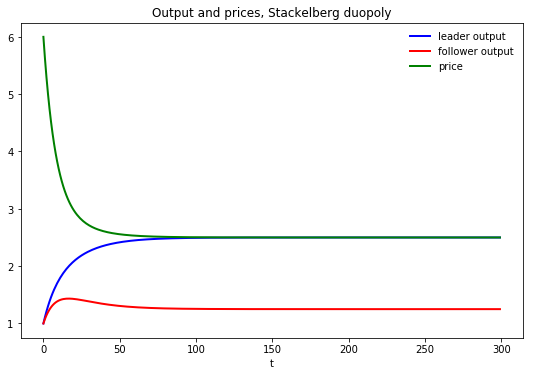We’ll compute the present value earned by the Stackelberg leader

We’ll compute it two ways (they give identical answers – just a check on coding and thinking)

In :
v_leader_forward = np.sum(βs * π_leader)
v_leader_direct = -yt[:, 0].T @ P @ yt[:, 0]

# == Display values == #
print("Computed values for the Stackelberg leader at t=0:\n")

Computed values for the Stackelberg leader at t=0:


In :
# Manually checks whether P is approximately a fixed point
P_next = (R + F.T @ Q @ F + β * (A - B @ F).T @ P @ (A - B @ F))
(P - P_next < tol0).all()

Out:
True
In :
# Manually checks whether two different ways of computing the
# value function give approximately the same answer
v_expanded = -((y0.T @ R @ y0 + ut[:, 0].T @ Q @ ut[:, 0] +
β * (y0.T @ (A - B @ F).T @ P @ (A - B @ F) @ y0)))
(v_leader_direct - v_expanded < tol0)[0, 0]

Out:
True

Exhibiting time inconsistency of Stackelberg plan¶

In the code below we compare two values

• the continuation value $- y_t P y_t$ earned by a continuation Stackelberg leader who inherits state $y_t$ at $t$
• the value of a reborn Stackelberg leader who inherits state $z_t$ at $t$ and sets $x_t = - P_{22}^{-1} P_{21}$

The difference between these two values is a tell-tale time of the time inconsistency of the Stackelberg plan

In :
# Compute value function over time with reset at time t

yt_reset = yt.copy()
yt_reset[-1, :] = (H_0_0 @ yt[:3, :])

for t in range(n):
vt_leader[t] = -yt[:, t].T @ P @ yt[:, t]
vt_reset_leader[t] = -yt_reset[:, t].T @ P @ yt_reset[:, t]

In :
fig, axes = plt.subplots(3, 1, figsize=(10, 7))

axes.plot(range(n+1), (- F @ yt).flatten(), 'bo', label='Stackelberg leader', ms=2)
axes.plot(range(n+1), (- F @ yt_reset).flatten(), 'ro', label='continuation leader at t', ms=2)
axes.set(title=r'Leader control variable $u_{t}$', xlabel='t')
axes.legend()

axes.plot(range(n+1), yt[3, :], 'bo', ms=2)
axes.plot(range(n+1), yt_reset[3, :], 'ro', ms=2)
axes.set(title=r'Follower control variable $x_{t}$', xlabel='t')

axes.set(title=r'Leader value function $v(y_{t})$', xlabel='t')

plt.tight_layout()
plt.show()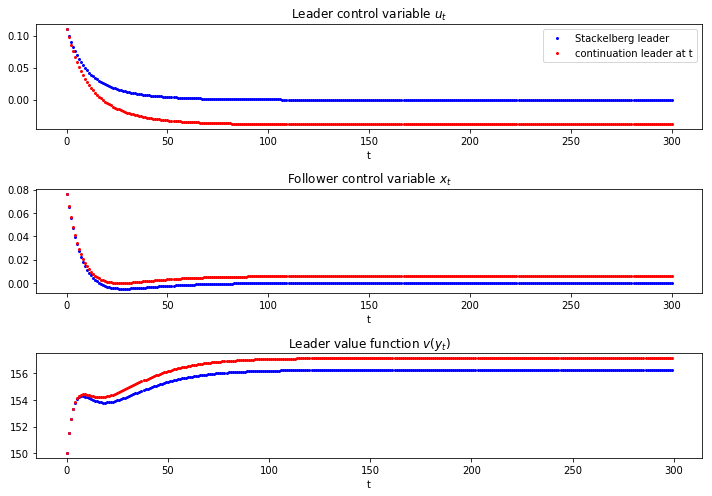Recursive formulation of the follower’s problem¶

We now formulate and compute the recursive version of the follower’s problem

We check that the recursive Big $K$ , little $k$ formulation of the follower’s problem produces the same output path $\vec q_1$ that we computed when we solved the Stackelberg problem

In :
A_tilde = np.eye(5)
A_tilde[:4, :4] = A - B @ F

R_tilde = np.array([[0,            0, 0,    0, -a0 / 2],
[0,            0, 0,    0,  a1 / 2],
[0,            0, 0,    0,       0],
[0,            0, 0,    0,       0],
[-a0 / 2, a1 / 2, 0,    0,      a1]])

Q_tilde = Q
B_tilde = np.array([[0, 0, 0, 0, 1]]).T

lq_tilde = LQ(Q_tilde, R_tilde, A_tilde, B_tilde, beta=β)
P_tilde, F_tilde, d_tilde = lq_tilde.stationary_values(method='doubling')

y0_tilde = np.vstack((y0, y0))
yt_tilde = lq_tilde.compute_sequence(y0_tilde, ts_length=n)

In :
# Checks that the recursive formulation of the follower's problem gives
# the same solution as the original Stackelberg problem
plt.plot(yt_tilde, 'r', label="q_tilde")
plt.plot(yt_tilde, 'b', label="q")
plt.legend()
plt.show()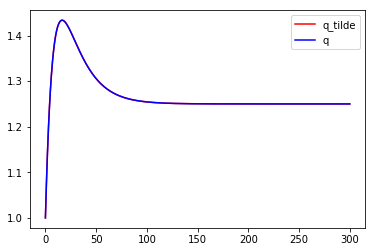Note: Variables with _tilde are obtained from solving the follower’s problem – those without are from the Stackelberg problem

In :
# Maximum absolute difference in quantities over time between the first and second solution methods
np.max(np.abs(yt_tilde - yt_tilde))

Out:
6.661338147750939e-16
In :
# x0 == x0_tilde
yt[:, 0][-1] - (yt_tilde[:, 1] - yt_tilde[:, 0])[-1] < tol0

Out:
True

Explanation of alignment¶

If we inspect the coefficients in the decision rule $- \tilde F$, we can spot the reason that the follower chooses to set $x_t = \tilde x_t$ when it sets $x_t = - \tilde F X_t$ in the recursive formulation of the follower problem

Can you spot what features of $\tilde F$ imply this?

Hint: remember the components of $X_t$

In :
# Policy function in the follower's problem
F_tilde.round(4)

Out:
array([[-0.    ,  0.    , -0.1032, -1.    ,  0.1032]])
In :
# Value function in the Stackelberg problem
P

Out:
array([[  963.54083615,  -194.60534465,  -511.62197962, -5258.22585724],
[ -194.60534465,    37.3535753 ,    81.97712513,   784.76471234],
[ -511.62197962,    81.97712513,   247.34333344,  2517.05126111],
[-5258.22585724,   784.76471234,  2517.05126111, 25556.16504097]])
In :
# Value function in the follower's problem
P_tilde

Out:
array([[-1.81991134e+01,  2.58003020e+00,  1.56048755e+01,
1.51229815e+02, -5.00000000e+00],
[ 2.58003020e+00, -9.69465925e-01, -5.26007958e+00,
-5.09764310e+01,  1.00000000e+00],
[ 1.56048755e+01, -5.26007958e+00, -3.22759027e+01,
-3.12791908e+02, -1.23823802e+01],
[ 1.51229815e+02, -5.09764310e+01, -3.12791908e+02,
-3.03132584e+03, -1.20000000e+02],
[-5.00000000e+00,  1.00000000e+00, -1.23823802e+01,
-1.20000000e+02,  1.43823802e+01]])
In :
# Manually check that P is an approximate fixed point
(P  - ((R + F.T @ Q @ F) + β * (A - B @ F).T @ P @ (A - B @ F)) < tol0).all()

Out:
True
In :
# Compute P_guess using F_tilde_star
F_tilde_star = -np.array([[0, 0, 0, 1, 0]])
P_guess = np.zeros((5, 5))

for i in range(1000):
P_guess = ((R_tilde + F_tilde_star.T @ Q @ F_tilde_star) +
β * (A_tilde - B_tilde @ F_tilde_star).T @ P_guess
@ (A_tilde - B_tilde @ F_tilde_star))

In :
# Value function in the follower's problem
-(y0_tilde.T @ P_tilde @ y0_tilde)[0, 0]

Out:
112.65590740578058
In :
# Value function with P_guess
-(y0_tilde.T @ P_guess @ y0_tilde)[0, 0]

Out:
112.6559074057807
In :
# Compute policy using policy iteration algorithm
F_iter = (β * la.inv(Q + β * B_tilde.T @ P_guess @ B_tilde)
@ B_tilde.T @ P_guess @ A_tilde)

for i in range(100):
# Compute P_iter
P_iter = np.zeros((5, 5))
for j in range(1000):
P_iter = ((R_tilde + F_iter.T @ Q @ F_iter) + β *
(A_tilde - B_tilde @ F_iter).T @ P_iter @
(A_tilde - B_tilde @ F_iter))

# Update F_iter
F_iter = (β * la.inv(Q + β * B_tilde.T @ P_iter @ B_tilde)
@ B_tilde.T @ P_iter @ A_tilde)

dist_vec = (P_iter - ((R_tilde + F_iter.T @ Q @ F_iter) +
β * (A_tilde - B_tilde @ F_iter).T @ P_iter @
(A_tilde - B_tilde @ F_iter)))

if np.max(np.abs(dist_vec)) < 1e-8:
dist_vec2 = (F_iter - (β * la.inv(Q + β * B_tilde.T @ P_iter @ B_tilde)
@ B_tilde.T @ P_iter @ A_tilde))

if np.max(np.abs(dist_vec)) < 1e-8:
F_iter
else:
print("The policy didn't converge: try increasing the number of outer loop iterations")
else:
print("P_iter didn't converge: try increasing the number of inner loop iterations")

In :
# Simulate the system using F_tilde_star and check that it gives the same result as the original solution

yt_tilde_star = np.zeros((n, 5))
yt_tilde_star[0, :] = y0_tilde.flatten()

for t in range(n-1):
yt_tilde_star[t+1, :] = (A_tilde - B_tilde @ F_tilde_star) @ yt_tilde_star[t, :]

plt.plot(yt_tilde_star[:, 4], 'r', label="q_tilde")
plt.plot(yt_tilde, 'b', label="q")
plt.legend()
plt.show()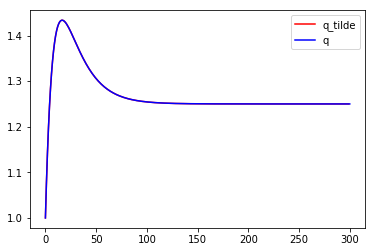In :
# Maximum absolute difference
np.max(np.abs(yt_tilde_star[:, 4] - yt_tilde[2, :-1]))

Out:
0.0

Markov perfect equilibrium¶

The state vector is

$$z_t = \begin{bmatrix} 1 \cr q_{2t} \cr q_{1t} \end{bmatrix}$$

and the state transition dynamics are

$$z_{t+1} = A z_t + B_1 v_{1t} + B_2 v_{2t}$$

where $A$ is a $3 \times 3$ identity matrix and

$$B_1 = \begin{bmatrix} 0 \cr 0 \cr 1 \end{bmatrix} , \quad B_2 = \begin{bmatrix} 0 \cr 1 \cr 0 \end{bmatrix}$$

The Markov perfect decision rules are

$$v_{1t} = - F_1 z_t , \quad v_{2t} = - F_2 z_t$$

and in the Markov perfect equilibrium the state evolves according to

$$z_{t+1} = (A - B_1 F_1 - B_2 F_2) z_t$$
In :
# == In LQ form == #
A = np.eye(3)
B1 = np.array([, , ])
B2 = np.array([, , ])

R1 = np.array([[0,            0, -a0 / 2],
[0,            0,  a1 / 2],
[-a0 / 2, a1 / 2,      a1]])

R2 = np.array([[0,       -a0 / 2,      0],
[-a0 / 2,      a1, a1 / 2],
[0,        a1 / 2,      0]])

Q1 = Q2 = γ
S1 = S2 = W1 = W2 = M1 = M2 = 0.0

# == Solve using QE's nnash function == #
F1, F2, P1, P2 = qe.nnash(A, B1, B2, R1, R2, Q1,
Q2, S1, S2, W1, W2, M1,
M2, beta=β, tol=tol1)

# == Simulate forward == #
AF = A - B1 @ F1 - B2 @ F2
z = np.empty((3, n))
z[:, 0] = 1, 1, 1
for t in range(n-1):
z[:, t+1] = AF @ z[:, t]

# == Display policies == #
print("Computed policies for firm 1 and firm 2:\n")
print(f"F1 = {F1}")
print(f"F2 = {F2}")

Computed policies for firm 1 and firm 2:

F1 = [[-0.22701363  0.03129874  0.09447113]]
F2 = [[-0.22701363  0.09447113  0.03129874]]

In :
q1 = z[1, :]
q2 = z[2, :]
q = q1 + q2       # Total output, MPE
p = a0 - a1 * q   # Price, MPE

fig, ax = plt.subplots(figsize=(9, 5.8))
ax.plot(range(n), q, 'b-', lw=2, label='total output')
ax.plot(range(n), p, 'g-', lw=2, label='price')
ax.set_title('Output and prices, duopoly MPE')
ax.legend(frameon=False)
plt.xlabel('t')
plt.show()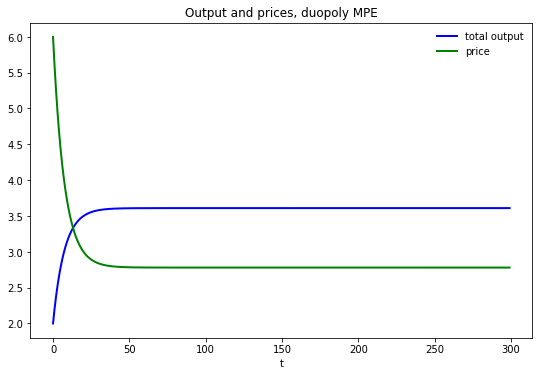In :
# Computes the maximum difference between the two quantities of the two firms
np.max(np.abs(q1 - q2))

Out:
6.8833827526759706e-15
In :
# Compute values
u1 = (- F1 @ z).flatten()
u2 = (- F2 @ z).flatten()

π_1 = p * q1 - γ * (u1) ** 2
π_2 = p * q2 - γ * (u2) ** 2

v1_forward = np.sum(βs * π_1)
v2_forward = np.sum(βs * π_2)

v1_direct = (- z[:, 0].T @ P1 @ z[:, 0])
v2_direct = (- z[:, 0].T @ P2 @ z[:, 0])

# == Display values == #
print("Computed values for firm 1 and firm 2:\n")
print(f"v1(forward sim) = {v1_forward:.4f}; v1 (direct) = {v1_direct:.4f}")
print(f"v2 (forward sim) = {v2_forward:.4f}; v2 (direct) = {v2_direct:.4f}")

Computed values for firm 1 and firm 2:

v1(forward sim) = 133.3303; v1 (direct) = 133.3296
v2 (forward sim) = 133.3303; v2 (direct) = 133.3296

In :
# Sanity check
Λ1 = A - B2 @ F2
lq1 = qe.LQ(Q1, R1, Λ1, B1, beta=β)
P1_ih, F1_ih, d = lq1.stationary_values()

v2_direct_alt = - z[:, 0].T @ lq1.P @ z[:, 0] + lq1.d

(np.abs(v2_direct - v2_direct_alt) < tol2).all()

Out:
True

MPE vs. Stackelberg¶

In :
vt_MPE = np.zeros(n)
vt_follower = np.zeros(n)

for t in range(n):
vt_MPE[t] = -z[:, t].T @ P1 @ z[:, t]
vt_follower[t] = -yt_tilde[:, t].T @ P_tilde @ yt_tilde[:, t]

plt.plot(vt_MPE, 'b', label='MPE')
plt.plot(vt_follower, 'g', label='Stackelberg follower')
plt.title(r'MPE vs. Stackelberg Value Function')
plt.xlabel('t')
plt.legend(loc=(1.05, 0))
plt.show()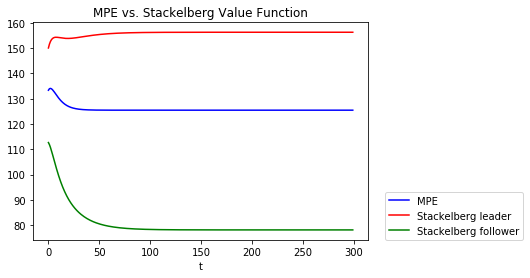In :
# == Display values == #
print("Computed values:\n")
print(f"vt_follower(y0) = {vt_follower:.4f}")
print(f"vt_MPE(y0) = {vt_MPE:.4f}")

Computed values:


# Compute the difference in total value between the Stackelberg and the MPE

-3.970942562087714